### IMO Shortlist 2006 problem G6

Kvaliteta:
Avg: 0,0
Težina:
Avg: 8,0
Circles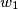$w_{1}$ and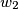$w_{2}$ with centres$O_{1}$ and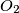$O_{2}$ are externally tangent at point$D$ and internally tangent to a circle$w$ at points$E$ and$F$ respectively. Line$t$ is the common tangent of$w_{1}$ and$w_{2}$ at$D$. Let$AB$ be the diameter of$w$ perpendicular to$t$, so that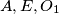$A, E, O_{1}$ are on the same side of$t$. Prove that lines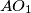$AO_{1}$,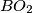$BO_{2}$,$EF$ and$t$ are concurrent.
Izvor: Međunarodna matematička olimpijada, shortlist 2006